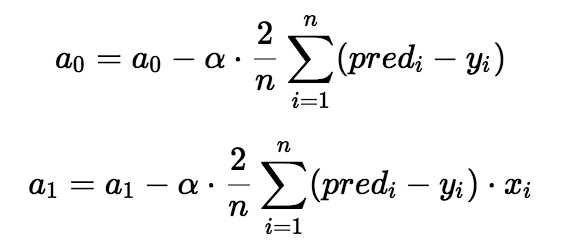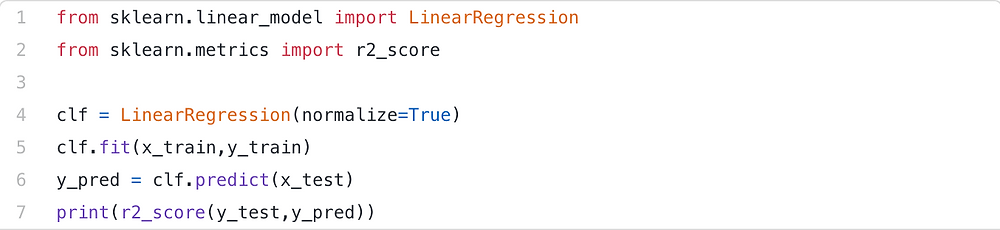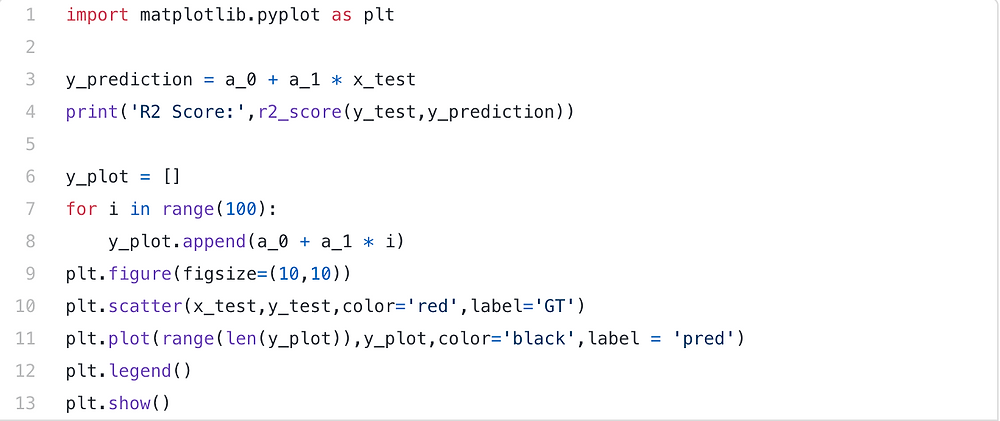Search

# Introduction to Machine Learning Algorithms: Linear Regression

Written by Satvik Tripathi

I recently attended Stanford Pre-Collegiate Summer Institute to study artificial intelligence and machine learning, taught by Ross Alexander. In this article, I’ll try to sum up some of the topics I learned during my time there.

Okie Doke, so let’s get started.

Recently Artificial Intelligence has become popular. People from different fields are trying to apply AI to make their tasks even simpler. For instance, economists use AI to predict future market prices to make a profit, doctors use AI to classify whether a tumor is malignant or benign, meteorologists use AI to predict the weather, HR recruiters use AI to check applicants' resumes to verify whether the applicant meets the minimum job criteria, etc. Machine-learning algorithms are the force behind the widespread use of AI. You are at the right place for someone who wants to know ML algorithms but still hasn't got their feet wet. The rudimentary algorithm with which any enthusiast in Machine Learning begins with is a linear regression algorithm. Therefore we must do the same as it provides us with a foundation for building on and studying other ML algorithms and ML models.

## What is Linear Regression?

Let us get used to regression until we learn what linear regression is. Regression is a method of modeling independent predictors to model a target value. This approach is often used for forecasting and finding out the relationship between variables between cause and effect. Regression methods vary mainly in terms of the number of independent variables and the form of relationship between the independent and dependent variables.Linear Regression

Simple linear regression is a type of regression analysis in which the number of independent variables is one and the independent (x) and dependent (y) variables are linearly related. In the graph above the red line is referred to as the line of best fit. Based on the given data points, we try to plot a line that models the points the best. Using the linear equation shown below, we model the line.

## Y = α + β X ()

```y = a_0 + a_1 * x   ## But I'll use a_0 and a_1 for the ease of writing

```

The purpose of the linear regression algorithm is to find the best values for both a_0 and a_1. Before we move on to the algorithm, let's look at two important concepts you need to learn to better understand Linear Regression.

## Cost Function

The cost function lets us work out the best possible values for a_0 and a_1 which will output the best-fit line possible for the given data points. Because we want the best values for a_0 and a_1, this search problem is translated into a problem of minimization, where we want to reduce the error between the predicted value and the actual value.Minimization and Cost Function

To minimize that, we choose the above function. The variation between predicted values and ground truth measures the error difference. We square the error difference and sum over all data points and divide that value by the total number of data points. This gives the squared error average for all the data points. The cost function is therefore also known as the Mean Squared Error (MSE) function. Now we're going to adjust the values of a_0 and a_1 by using this MSE function so that the MSE value settles at the minima.

The next important term that is required to understand linear regression is gradient descent. Gradient descent is a method to update a_0 and a_1 to minimize cost function (MSE). The concept is that we randomly start with some values for a_0 and a_1 and then iteratively adjust those values to reduce the cost. Gradient descent helps us to swiftly change values.To draw an analogy, imagine a U-shaped pit and you stand at the top of the pit and your objective is to get to the bottom of the pit. There is a catch, only a discrete number of steps can be taken to get to the edge. If you want to take one move at a time, you will finally hit the pit's edge, but it will take longer. When you want to take longer steps each time, you'd hit faster, but there's a risk you'd be able to overshoot the pit's bottom and not quite down. The number of steps you take in the gradient descent algorithm is the learning rate. This determines how quickly the algorithm converges to the minima.A Visual Representation of Gradient Decent

Often the cost function can be a non-convex function where you can settle to a local minima but it's still a convex function for linear regression.

You may wonder how to optimize a_0 and a_1 using gradient descent. To update a_0 and a_1, we take gradients from the cost function. We take partial derivatives with respect to a_0 and a_1 to find these gradients. Now, you 'd need some calculus to understand how the partial derivatives are found below, but if you don't, it's totally okay. You can take it as it is.Which gives us-The partial derivatives are the gradients, which are used to change a_0 which a_1 values. Alpha is the learning rate, a hyperparameter, and you must specifically determine it. A lower rate of learning could bring you closer to the minima, but it takes more time to reach the minima, a higher rate of learning converges sooner, but there is a chance you could overshoot the minima.

We will post a separate article covering only gradient descent and it will give you a better understanding of this algorithm.

## Code

Let's get to my favorite part, coding. We have two choices; either we can use the scikit learn library to import and directly use the linear regression model or we can write our own regression model based on the above equations. Rather of going for one of the two, let's do both :)

There are numerous datasets available online for linear regression. I used the one from this link. Let’s visualize the training and testing data.Training DataTesting Data

Let's start with the easiest of the two methods, i.e. to build our linear regression model using scikit learn library.We use the pandas library to read the train files and to test them. We retrieve the independent (x) and dependent (y) variables and because we only have one feature (x) we have to reshape them so we can insert them into our linear regression model.Now, We’ll use scikit learn to import the linear regression model (there are enormous numbers of scikit learn models to play with). We fit the model on the training data and predict the values for the testing data. We use the R2 score to assess our model’s accuracy.R2 score on Testing Data

Now let's develop our own model for linear regression from the above equations. We'll use the NumPy library for the computations and the metric's R2 score.We initialize both a_0 and a_1 with the value 0.0. We calculate the cost for 1000 epochs (iterations), and using the cost we calculate the gradients and update the values of a_0 and a_1 using the gradients. We would have obtained the best values for a_0 and a_1 after 1000 epochs and therefore we can formulate the best fit line.The test set includes 300 samples, so we need to reshape the a_0 and a_1 from 700x1 to 300x1. Now, we can use the equation only to estimate values in the test set and get the R2 score.R2 score on Testing Data(using Numpy)

As with the previous approach, we can observe the same R2 value. We also map the line of regression along with the test data points to get a better visual understanding of how well our algorithm is performing.Regression line — Test data

This looks Perfect!

## Conclusion

Linear Regression is an algorithm that Machine Learning enthusiasts or practitioners must know and it's also the best starting point for people who also want to learn Machine Learning. It's a simple but useful algorithm. With more such content, I hope I could get you till the end of this article and hope that you’d implement some of what you learned/understood today in your day to day programming and machine learning. Until then, stay enthusiastic and motivated. Okie Doke, see you next time!

REFERENCES:

https://medium.com/@jagwithyou/machine-learning-tutorials-96aef4c44f50